# Configuration Keys¶

The following sections give all possible configuration keys that may be used in your .conf file to configure your fitting run. Each line of the .conf file sets the value of a configuration key with the general syntax:

```key = value
```

## Required Keys¶

model

Specifies the mapping between model files (.bngl or .xml) and data files (.exp or .prop). Model paths and files are followed by a ‘:’ and then a comma-delimited list of experimental data files or property files corresponding to the model files. If no experimental files are associated with a model write `none` instead of a file path.

Examples:

• `model = path/to/model1.bngl : path/to/data1.exp`
• `model = path/to/model2.xml : path/to/data2.prop, path/to/data2.exp`
• `model = path/to/model3.xml : none`
fit_type

The choice of fitting algorithm. Options:

Example:

• `fit_type = de`
objfunc

Which objective function to use.

• `chi_sq` - Chi squared
• `chi_sq_dynamic` - Chi squared with sigma as a free parameter (Requires sigma__FREE in the model and the configuration file)
• `neg_bin` - Negative Binomial (Requires neg_bin_r set to a number in the configuration file i.e neg_bin_r = 2, Default = 24)
• `neg_bin_dynamic` - Negative Binomial with r as a free parameter (Requires r__FREE in the model and the configuration file)
• `kl` - Kullback-Leibler
• `sos` - Sum of squares
• `sod` - Sum of differences
• `norm_sos` - Sum of squares, normalized by the value at each point,
• `ave_norm_sos` - Sum of squares, normalized by the average value of the variable.

Default: chi_sq

Example:

• `objfunc = chi_sq`
population_size

The number parameter sets to maintain in a single iteration of the algorithm. See algorithm descriptions for more information.

Example:

• `population_size = 50`
max_iterations

Maximum number of iterations

Example:

• `max_iterations = 200`

## Other Path Keys¶

bng_command

Path to BNG2.pl, including the BNG2.pl file name. This key is required if your fitting includes any .bngl files, unless the BioNetGen path is specified with the BNGPATH env variable.

Default: Uses the BNGPATH environmental variable

Example:

• `bng_command = path/to/BNG2.pl`
output_dir

Directory where we should save the output.

Default: “pybnf_output”

Example:

• `output_dir = dirname`

## Parameter and Model Specification¶

mutant

Declares a model that does not have its own model file, but instead is defined based on another model (the “base model”), changing only a small number of parameter values. The first word of the declaration gives the name of the base model (not including the path or .bngl/.xml extension). The second word is the name of the mutant model; this name is appended to the suffixes of the base model. That is, if the base model has data files `data1.exp` and `data2.exp`, a corresponding mutant model with the name “m1” should use the files `data1m1.exp` and `data2m1.exp`. Following the name of the mutant model is a series of statements that specify how to change `basemodel` to make the mutant model. The statements have the format [variable][operator][value] ; for example `a__FREE=0` or `b__FREE*2`. Supported operators are `=`, `+`, `-`, `*`, `/`.

Default: None

Example:

Elsewhere in your .conf file, you have specified model1:

• `model = path/to/model1.bngl : data1.exp`

Then you can use this key as follows:

• `mutant = model1 no_a a__FREE=0 : data1no_a.exp, data2no_a.exp`
• `mutant = model1 extra_ab a__FREE*2 b__FREE*2 : data1extra_ab.exp`
uniform_var

A bounded uniformly distributed variable defined by a 3-tuple corresponding to the variable name, minimum value, and maximum value. If the tag `U` is added to the end, the bounds are enforced only during initialization, not during fitting.

Examples:

• `uniform_var = k__FREE 10 20`
• `uniform_var = k__FREE 10 20 U`
normal_var

A normally distributed variable defined by a 3-tuple: the name, mean value, and standard deviation. The distribution is truncated at 0 to prevent negative values

Example:

• `normal_var = d__FREE 10 1`
loguniform_var

A variable distributed uniformly in logarithmic space. The value syntax is identical to the uniform_var syntax

Examples:

• `loguniform_var = p__FREE 0.001 100`
• `loguniform_var = p__FREE 0.001 100 U`
lognormal_var

A variable normally distributed in logarithmic space. The value syntax is a 3-tuple specifying the variable name, the base 10 logarithm of the mean, and the base 10 logarithm of the standard deviation

Example:

• `lognormal_var = l__FREE 1 0.1`

The following two keys (`var` and `logvar`) are to be used only with the simplex algorithm. Simplex should not use any of the other parameter specifications. If you are using another algorithm with the flag `refine`, you must set the simplex algorithm’s parameters with `simplex_step` or `simplex_log_step`.

var

The starting point for a free parameter. It is defined by a 3-tuple, corresponding to the variable’s name, its initial value and an initial step size (optional). If not specified, the initial step size defaults to the value specified by the simplex-specific parameter `simplex_step` (see simplex)

Examples:

• `var = k__FREE 10`
• `var = d__FREE 2 0.05`
logvar

Syntax and sematics are identical to the `var` key above, but the initial value and initial step should be specified in base 10 logarithmic space.

Example:

• `logvar = k__FREE -3 1`

## Simulation Actions¶

These keys specify what simulations should be performed with the models. For SBML models, simulation actions are required. For BNGL models, the same information can be specified in the actions block of the BNGL file, so use of these keys is optional.

time_course

Run a time course simulation on the model. Specify a comma-delimited list of `key:value` pairs, with the following possible keys:

• `time`: The simulation time. Required.

• `suffix`: The suffix of the data file to save. You should map the model to a .exp file of the same name. Default: time_course

• `step`: The simulation time step. Default: 1

• `model`: The name of the model to run (not including the path or .bngl/.xml extension). Default: All models in the fitting run.

• `subdivisions`: Only for use with `sbml_integrator=euler`, specifies the number of internal Euler steps to perform between each output step specified by `step`. Default: 1

• `method` The simulation method to use. Default is `ode`. Options are:

• `ode`: Numerical integration of differential equations
• `ssa`: Stochastic simulation algorithm (BioNetGen’s “ssa” algorithm for BNGL models; Gillespie’s direct method for SBML models)
• `pla`: Partitioned-leaping algorithm (BNGL models only)
• `nf`: Network-free simulation with NFsim (BNGL models only)

Example:

• `time_course = time:60, model:model1, suffix:data1`
param_scan

Run a parameter scan on the model. Specify a comma-delimited list of `key:value` pairs, with the following possible keys:

• `param`: Name of the parameter to scan. Required.
• `min`: Minimum value of the parameter. Required
• `max`: Maximum value of the parameter. Required.
• `step`: Change in the parameter value between consecutive simulations in the scan. Required.
• `time`: The simulation time. Required.
• `suffix`: The suffix of the data file to save. You should map the model to a .exp file of the same name. Default: param_scan
• `logspace`: If 1, take `step` to be in log (base 10) space, and scan the parameter in log (base 10) space. Default: 0
• `model`: The name of the model to run (not including the path or .bngl/.xml extension). Default: All models in the fitting run.
• `subdivisions`: Only for use with `sbml_integrator=euler`, specifies the number of internal Euler steps to perform for each simulation. Default: 1000
• `method`: The simulation method to use. Options are the same as in `time_course`. Default: ode

Example:

• `param_scan = param:x, min:1, max:1000, step:0.5, logspace:1, time:60, model:model1, suffix:data1`

## Parallel Computing¶

parallel_count

The number of jobs to run in parallel. This may be set for both local and cluster fitting runs. For cluster runs, this number is divided by the number of available nodes (and rounded up) to determine the number of parallel jobs per node.

Default: Use all available cores. On a cluster, the number of available cores per node is determined by running `multiprocessing.cpu_count()` from the scheduler node.

Example:

• `parallel_count = 7`
cluster_type

Type of cluster used for running the fit. This key may be omitted, and instead specified on the command line with the `-t` flag. Currently supports `slurm` or `none`.

Default: None (local fitting run).

Example:

• `cluster_type = slurm`
parallelize_models

For fitting jobs that include multiple models, run those models on different cores, utilizing a total of this number of cores per parameter set evaluation. Should not be set higher than the total number of models. Using this option incurs additional communication overhead, and causes the objective function to be evaluated locally, not in parallel. Therefore, only certain types of problems will benefit from this option.

Default: 1

Example:

• `parallelize_models = 3`
scheduler_file

Provide a scheduler file to link PyBNF to a Dask scheduler already created outside of PyBNF. See Manual configuration with Dask for more information. This option may also be specified on the command line with the `-s` flag.

Default: None

Example:

• `scheduler_file = cluster.json`
scheduler_node

Manually set node used for creating the distributed Client – takes a string identifying a machine on a network. If running on a cluster with SLURM, it is recommended to use automatic configuration with the flag `-t slurm` instead of using this key.

Default: None

Example:

• `scheduler_node = cn180`
simulation_dir

Optional setting for a different directory where we should save (or temporarily store) simulation output. Usually not necessary to set separately from output_dir. However, if you are running on a cluster with a Lustre filesystem, you may want to set this to a different disk to avoid excessive reads and writes to the Lustre disk.

Default: Use the same directory as output_dir.

Example:

• `simulation_dir = /scratch/sim_output`
worker_nodes

Manually set nodes used for computation - takes one or more strings separated by whitespace identifying machines on a network. If running on a cluster with SLURM, it is recommended to use automatic configuration with the flag `-t slurm` instead of using this key.

Default: None

Example:

• `worker_nodes = cn102 cn104 cn10511`

## General Options¶

### Output Options¶

delete_old_files

Takes an integer for a value. If 1, delete simulation folders immediately after they complete. If 2, delete both old simulation folders and old sorted_params.txt result files. If 0, do not delete any files (warning, could consume a large amount of disk space).

Default: 1

Example:

• `delete_old_files = 2`
num_to_output

The maximum number of parameter sets to output when writing the trajectory to file. The parameter sets are ordered by their corresponding objective function value to ensure the best fits are outputted.

Default: 5000

Example:

• `num_to_output = 100000`
output_every

The number of iterations in between consecutive events writing the trajectory to file.

Default: 20

Example:

• `output_every = 1000`
save_best_data

If 1, run an extra simulation at the end of fitting using the best-fit parameters, and save the best-fit .gdat and .scan files to the Results directory.

Default: 0

Example:

• `save_best_data = 1`
verbosity

An integer value that specifies the amount of information output to the terminal.

• 0 - Quiet: User prompts and errors only
• 1 - Normal: Warnings and concise progress updates
• 2 - Verbose: Information and detailed progress updates

Default: 1

Example:

• `verbosity = 0`

### Algorithm Options¶

bootstrap

If assigned a positive value, estimate confidence intervals through a bootstrapping procedure. The assigned integer is the number of bootstrap replicates to perform.

Default: 0 (no bootstrapping)

Example:

• `bootstrap = 10`
bootstrap_max_obj

The maximum value of a fitting run’s objective function to be considered valid in the bootstrapping procedure. If a fit ends with a larger objective value, it is discarded.

Default: None

Example:

• `bootstrap_max_obj = 1.5`
constraint_scale

Scale all weights in all .prop files by this multiplicative factor. For convenience only - The same thing could be achieved by editing .prop files, but this option is useful for tuning the relative contributions of quantitative and qualitative data.

Default: 1 (no scaling)

Example:

• `constraint_scale = 1.5`
ind_var_rounding

If 1, make sure every exp row is used by rounding it to the nearest available value of the independent variable in the simulation data. (Be careful with this! Usually, it is better to set up your simulation so that all experimental points are hit exactly)

Default: 0

Example:

• `ind_var_rounding = 1`
initialization

How to initialize parameters.

• `rand` - initialize params randomly according to the distributions.
• `lh` - For `random_var`s and `loguniform_var`s, initialize with a latin hypercube distribution, to more uniformly cover the search space.

Default: lh

Example:

• `initialization = rand`
local_objective_eval

If 1, evaluate the objective function locally, instead of parallelizing this calculation on the workers. This option is automatically enabled when using the `smoothing` or `parallelize_models` feature.

Default: 0 (unless smoothing is enabled)

Example:

• `local_objective_eval = 1`
min_objective

Stop fitting if an objective function lower than this value is reached.

Default: None; always run for the maximum iterations

Example:

• `min_objective = 0.01`
normalization

Indicates that simulation data must be normalized in order to compare with exp files. Specify one of the following types of normalization:

• `init` - normalize to the initial value
• `peak` - normalize to the maximum value
• `zero` - normalize such that each column has a mean of 0 and a standard deviation of 1
• `unit` - Scales data so that the range of values is between (min-init)/(max-init) and 1 (if the maximum value is 0 (i.e. max == init), then the data is scaled by the minimum value after subtracting the initial value so that the range of values is between 0 and -1).

If only the type is specified, the normalization is applied to all exp files. If the type is followed by a ‘:’ and a comma-delimited list of exp files, it applies to only those exp files. Additionally, you may enclose an exp file in parentheses, and specify which columns of that exp file get normalized, as in `(data1.exp: 1,3-5)` or `(data1.exp: var1,var2)`. Multiple lines with this key can be used.

Default: No normalization

Examples:

• `normalization = init`
• `normalization = init: data1.exp, data2.exp`
• `normalization = init: (data1.exp: 1,3-5), (data2.exp: var1,var2)`
postprocess

Used to specify a custom Python script for postprocessing simulation results before evaluating the objective function. Specify the path to the Python script, followed by a list of all of the simulation suffixes for which that postprocessing script should be applied. For how to set up a postprocessing script, see Custom Postprocessing.

Default: No postprocessing

Example:

• `postprocess = path/to/script.py suff1 suff2`
refine

If 1, after fitting is completed, refine the best fit parameter set by a local search with the simplex algorithm.

Default: 0

Example:

• `refine = 1`
sbml_integrator

Which integrator to use for SBML models. Options are `cvode`, `rk4`, `gillespie`, or `euler`, and are described in the libroadrunner documentation. If your `time_course` or `param_scan` key specifies `method: ssa`, then `gillespie` is used for that action, overriding this setting.

Default: cvode

Example:

• `sbml_integrator = rk4`
smoothing

Number of replicate runs to average together for each parameter set (useful for stochastic simulations).

Default: 1

Example:

• `smoothing = 2`
wall_time_gen

Maximum time (in seconds) to wait to generate the network for a BNGL model. Will cause the program to exit if exceeded.

Default: 3600

Example:

• `wall_time_gen = 600`
wall_time_sim

Maximum time (in seconds) to wait for a simulation to finish. Exceeding this results in an infinite objective function value. Caution: For SBML models, using this option has an overhead cost, so only use it when needed.

Default: 3600 for BNGL models; No limit for SMBL models

Example:

• `wall_time_sim = 600`

## Algorithm-specific Options¶

### Simplex¶

These settings for the simplex algorithm may also be used when running other algorithms with `refine = 1`.

simplex_step

In initialization, we perturb each parameter by this step size. If you specify a step size for a specific variable via `var` or `logvar`, it overrides this setting.

Default: 1

Example:

• `simplex_step = 0.5`
simplex_log_step

Equivalent of `simplex_step`, for variables that move in log space.

Default: Value of `simplex_step`

Example:

• `simplex_log_step = 0.5`
simplex_reflection

When we reflect a point through the centroid, what is the ratio of dilation on the other side?

Default: 1.0

Example:

• `simplex_reflection = 0.5`
simplex_expansion

If the reflected point was the global minimum, how far do we keep moving in that direction? (as a ratio to the initial distance to centroid)

Default: 1.0

Example:

• `simplex_expansion = 0.5`
simplex_contraction

If the reflected point was not an improvement, we retry at what distance from the centroid? (as a ratio of the initial distance to centroid)

Default: 0.5

Example:

• `simplex_contraction = 0.3`
simplex_shrink

If a whole iteration was unproductive, shrink the simplex by setting simplex point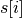to, where x is the value of this key and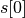is the best point in the simplex.

Default: 0.5

Example:

• `simplex_shrink = 0.3`
simplex_max_iterations

If specified, overrides the `max_iterations` setting. Useful if you are using the `refine` flag and want `max_iterations` to refer to your main algorithm.

Example:

• `simplex_max_iterations = 20`
simplex_stop_tol

Stop the algorithm if all parameters have converged to within this value (specifically, if all reflections in an iteration move the parameter by less than this value)

Default: 0 (don’t use this criterion)

Example:
• `simplex_stop_tol = 0.01`

### Differential Evolution¶

PyBNF offers two versions of differential evoltution: synchronous differential evolution (`fit_type = de`) and asynchronous differential evolution (`fit_type = ade`). Both versions may be configured with the follwing keys.

mutation_rate

When generating a new individual, mutate each parameter with this probability.

Default: 0.5

Example:

• `mutation_rate = 0.7`
mutation_factor

When mutating a parameter x, change it by mutation_factor*(PS1[x] - PS2[x]) where PS1 and PS2 are random other PSets in the population.

Default: 1.0

Example:

• `mutation_factor = 0.7`
stop_tolerance

Stop the run if within the current popluation,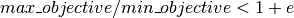, where e is the value of this key. This criterion triggers when the entire population has converged to roughly the same objective function value.

Default: 0.002

Example:

• `stop_tolerance = 0.001`
de_strategy

Specifies how new parameter sets are chosen. The following options are available:

• `rand1`
• `rand2`
• `best1`
• `best2`
• `all1`
• `all2`

The first part of the string determines which parameter set we mutate:

• `rand` - a random one
• `best` - the one with the lowest objective value
• `all` - the one we are proposing to replace (so all psets are mutated once per iteration).

The second part of the string specifies how we calculate the amount by which to mutate each parameter:

• `1` - Use 1 pair of other parameter sets: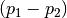• `2` - Use 2 pairs of other parameter sets: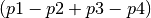.

Default: rand1

Example:

• `de_strategy = rand2`

The following options are only available with `fit_type = de`, and serve to make the algorithm more asynchronous. If used, these options enable island-based differential evolution, which is asynchronous in that each island can independently proceed to the next iteration.

islands

Number of separate populations to evolve.

Default: 1

Example:

• `islands = 2`
migrate_every

After this number of generations, migrate some individuals between islands.

Default: 20 (but Infinity if `islands = 1`)

Example:

• `migrate_every = 10`
num_to_migrate

How many individuals to migrate off of each island during migration.

Default: 3

Example:

• `num_to_migrate = 5`

### Particle Swarm¶

cognitive

Acceleration toward a particle’s own best fit

Default: 1.5

Example:

• `cognitive = 1.7`
social

Acceleration toward the global best fit

Default: 1.5

Example:

• `social = 1.7`
particle_weight

Inertia weight of particle. A value less than 1 can be thought of as friction that contniuously decelerates the particle.

Default: 0.7

Example:

• `particle_weight = 0.9`
v_stop

Stop the algorithm if the speeds of all parameters in all particles are less than this value.

Default: 0 (don’t use this criterion)

Example:

• `v_stop = 0.01`

A variant of particle swarm that adaptively changes the `particle_weight` over the course of the fitting run is configured with the following parameters. See the algorithm documentation for more information.

particle_weight_final

The final particle weight after the adaptive weight changing.

Default: the value of `particle_weight`, effectively disabling this feature.

Example:

• `particle_weight_final = 0.5`

After this many “unproductive” iterations, we have moved halfway from the initial weight to the final weight.

Default: 30

Example:

• `adaptive_n_max = 20`

Afer this many “unproductive” iterations, stop the fitting run.

Default: Inf

Example:

• `adaptive_n_stop = 50`

Parameter for checking if an iteration was “unproductive”

Default: 0

Example:

• `adaptive_abs_tol = 0.01`

Parameter for checking if an iteration was “unproductive”

Default: 0

Example:

• `adaptive_rel_tol = 0.01`

### Bayesian Algorithms (mh, pt, sa)¶

In the family of Bayesian algoritms with Metropolis sampling, PyBNF includes Metropolis-Hastings MCMC (`fit_type = mh`), Parallel Tempering (`fit_type = pt`), and Simulated Annealing (`fit_type = sa`). These algorithms have many configuration keys in common, as described below.

#### For all Bayesian algorithms¶

step_size

When proposing a Monte Carlo step, the step in n-dimensional parameter space has this length.

Default: 0.2

Example:

• `step_size = 0.5`
beta

Sets the initial beta (1/temperature). A smaller beta corresponds to a more broad exploration of parameter space. If a single value is provided, that beta is used for all replicates. If multiple values are provided, an equal number of replicates uses each value.

For `mh`, should be set to 1 (the default) to get the true probability distribution.

For `pt`, should specify multiple values: the number of values should equal `population_size`/`reps_per_beta`. Or you may instead use the `beta_range` key. Only the largest beta value in the list will constribute to statistical samples, and to get the true probability distribution, this maximum value should be 1.

For `sa`, should typically be set to a single, small value which will increase over the course of the fitting run.

Default: 1

Examples:

• `beta = 0.9`
• `beta = 0.7 0.8 0.9 1`

#### For all Bayesian algorithms except `sa`¶

sample_every

Every x iterations, save the current PSet into the sampled population. Default: 100

Example:

• `sample_every = 20`
burn_in

Don’t sample for this many iterations at the start, to let the system equilibrate.

Default: 10000

Example:

• `burn_in = 1000`
output_hist_every

Every x samples (i.e every x*sample_every iterations), save a historgram file for each parameter, and the credible interval files, based on what has been sampled so far. Regardless, we also output these files at the end of the run.

Default: 100

Example:

• `output_hist_every = 10`
hist_bins

Number of bins used when writing the histogram files.

Default: 10

Example:

• `hist_bins = 20`
credible_intervals

Specify one or more numbers here. For each n, the algorithm will save a file giving bounds for each parameter such that in n% of the samples, the parameter lies within the bounds.

Default: 68 95

Examples:

• `credible_intervals = 95`
• `credible_intervals = 20 68 95`

#### For Simulated Annealing¶

beta_max

Stop the algorithm if all replicates reach this beta (1/temperature) value.

Default: Infinity (don’t use this stop criterion)

Example:

• `beta_max = 1.5`
cooling

Each time a move to a higher energy state is accepted, increase beta (1/temperature) by this value.

Default: 0.01

Example:

• `cooling = 0.001`

#### For Parallel Tempering¶

exchange_every

Every x iterations, perform replica exchange, swapping replicas that are adjacent in temperature with a statistically correct probability

Default: 20

Example:

• `exchange_every = 10`
reps_per_beta

How many identical replicas to run at each temperature. Must be a divisor of `population_size`.

Default: 1

Example:

• `reps_per_beta = 5`
beta_range

As an alternative to setting `beta`, the range of values of beta to use. Specify the minimum value, followed by the maximum value. The replicates will use `population_size`/`reps_per_beta` geometrically spaced beta values within this range. Only the replicas at the max beta value will be sampled. For the true probability distribution, the maximum value should be 1.

Default: None (betas are set with the `beta` key)

Example:

• `beta_range = 0.5 1`

stablizingCov

Stabilize the covariant matrix of the proposal.

Default: 0.001

Example:

• `stablizingCov = 0.1`

The number of iterations that the simulation will spend collecting data to observe the data for calcualtion of the differential matrix.``.

Default: 10000

Example:

• `adaptive = 50000`
output_noise_trajectory (Only for use with neg_bin and neg_bin_dynamic functions)

Calculate and add the negative binomial noise to the specified observables or functions then save the output of the user defined observable or function from the simulation output to a .txt file.

Default: None (multiple values can be defined separated by a comma)

Note: output_trajectory and output_noise_trajectory can both be declared in the same configuration file but may result in slower performance

Example:

• `output_noise_trajectory = ObservableA`
• `output_noise_trajectory = ObservableA, ObservableB, FunctionA`
output_trajectory

Save the output of the user defined observable or function from the simulation output to a .txt file.

Default: None (multiple values can be defined separated by a comma)

Example:

• `output_trajectory = ObservableA`
• `output_trajectory = ObservableA, ObservableB, FunctionA`
continue_run

When set to 1 the chains began at the MAP parameters, calculated covarience matrix, and diffusivity from the previous chain.

Default: 0

Example:

• `continue_run = 1`
calculate_covari

Calculate the covairance matrix of a defined segment of the previous run

Default: None

Example:

• `calculate_covari = 1 50000`
starting_params

Seed the run from a defined set of starting parameters listed in the same order they are defined with a space seperating each value in the order they are listed as free parameters in the configuratuib file

Default: None

Example:

• `starting_params = 5.5 2 3`